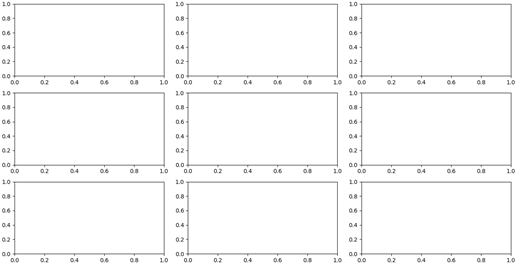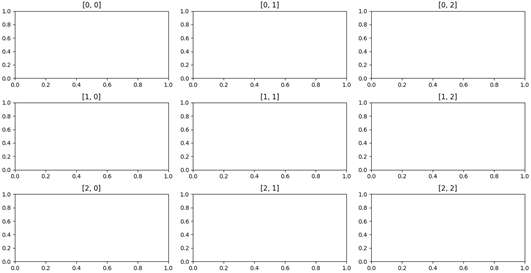# Matplotlib – How to Change Subplot Sizes

5/5 - (1 vote)

How to change the size of your `matplotlib` subplots?

The method `.subplots()` can easily generate multiple subplots within the same plotting figure. But as you may have already noticed, all the subplots have the exact same size and moreover, their size will be adjusted automatically, depending on how many of them you want to display in the same figure.

## Quick Solution Overview

To change the size of subplots in Matplotlib, use the `plt.subplots()` method with the `figsize` parameter (e.g., `figsize=(8,6)`) to specify one size for all subplots — unit in inches — and the `gridspec_kw` parameter (e.g., `gridspec_kw={'width_ratios': [2, 1]}`) to specify individual sizes.

Here’s a quick overview:

```# Set one size for all subplots
fig, ax = plt.subplots(2, 2, figsize=(8,6))

# Set individual sizes for specific subplots
fig, ax = plt.subplots(1, 2, gridspec_kw={'width_ratios': [2, 1]})```

In the next sections, you’ll learn how to change the size of each individual subplot. This is useful for tailoring the layout of your graphs and data presentations according to the relevance that you want to assign to each subplot.

## Simple Example figsize Argument

In the following example, I’ll show you how to use the `figsize` argument of the `plt.subplots()` method to change the size of a subplot:

```import matplotlib.pyplot as plt

# define subplots with subplot size
fig, ax = plt.subplots(2, 2, figsize=(8,6))

# define data
x = [0, 1, 2, 3, 4, 5]
y = [i**2 for i in x]

# create subplots
ax[0, 0].plot(x, y, color='black')
ax[0, 1].plot(x, y, color='green')
ax[1, 0].plot(x, y, color='red')
ax[1, 1].plot(x, y, color='blue')

# display the plot
plt.show()
```

Output:

Let’s change the `figsize` argument to a smaller tuple value:

• Original subplot: `fig, ax = plt.subplots(2, 2, figsize=(8,6))`
• Changed size: `fig, ax = plt.subplots(2, 2, figsize=(3,12))`
```import matplotlib.pyplot as plt

# define subplots
fig, ax = plt.subplots(2, 2, figsize=(3,12))

# define data
x = [0, 1, 2, 3, 4, 5]
y = [i**2 for i in x]

# create subplots
ax[0, 0].plot(x, y, color='black')
ax[0, 1].plot(x, y, color='green')
ax[1, 0].plot(x, y, color='red')
ax[1, 1].plot(x, y, color='blue')

# display the plot
plt.show()
```

Output — this strange looking beast:

This is if you want to change the size of all subplots at once.

## Simple Solution: Specifying Width Ratios Using subplots() gridspec_kw

The gridspec_kw parameter of the plt.subplot() function allows you to define a width relationship between individual subplots columns:

• `plt.subplots(2, 2, gridspec_kw={'width_ratios': [2, 1]})` sets the first column to double the size of the second column.
• `plt.subplots(2, 2, gridspec_kw={'width_ratios': [1, 2]})` sets the first column to half the size of the second column.

Here’s an example that draws from the previous code snippet:

```import matplotlib.pyplot as plt

# define subplots
fig, ax = plt.subplots(2, 2, gridspec_kw={'width_ratios': [2, 1]})

# define data
x = [0, 1, 2, 3, 4, 5]
y = [i**2 for i in x]

# create subplots
ax[0, 0].plot(x, y, color='black')
ax[0, 1].plot(x, y, color='green')
ax[1, 0].plot(x, y, color='red')
ax[1, 1].plot(x, y, color='blue')

# display the plot
plt.show()
```

Output:

You can see how the second column of subplots has only half the size of the first column of subplots as controlled by the `gridspec_kw` parameter.

The usual `.subplots()` method is really practical for creating multiple subplots but it is not able to access and change the size of these subplots.

On the other hand, the method `.add_gridspec()` results to be more time-consuming for just creating multiple subplots of the same size but, it constitutes a powerful solution when we want to change the size of individual subplots.

After subdividing the space in a grid-like fashion, you can use the method `.add_subplot()`, to change the size of each subplot by simply exploiting Python indexing and slicing.

## Slow Guide to Creating the Matplotlib Figure

In this example, we will only use the Matplotlib library for plotting the axes and for changing their size; for this reason, we will just import that specific package as shown before:

`import matplotlib.pyplot as plt`

We start our code by generating the plotting window, i.e. a figure, and then we will add some subplots to it.

If you are already familiar with the creation of multiple subplots, you can skip this section and go directly to the following one. If you instead do not know how to do that, or you simply forgot, it just takes a single code line.

The matplotlib function that is exploited for this aim is called `.figure()`.

This time, we also enter a property called `constrained_layout` and we set it equal to `True`.

This property can be used to fit the plots within the figure cleanly, meaning that they will automatically be nicely distributed in your plotting window, independently of their number.

We finally assign the figure to the variable “`fig`”.

`fig = plt.figure(constrained_layout=True)`

At this point, we have to create multiple subplots that we will then modify.

One of the most widely used methods for doing that is  `.subplots()`, which generates an array of axes, depending on the numbers that we enter as input parameters.

Suppose we want to create 9 subplots; it is sufficient to type:

```# Creating the subplots with .subplots()
ax = fig.subplots(3,3)```

These lines will yield 9 subplots, distributed in a 3×3 grid fashion, like the ones displayed in Figure 1.Figure 1: By using the method .subplots(3, 3)we are able to generate nine subplots, automatically distributed in a 3×3 grid fashion.

Achieving the same result using the method `.add_gridspec()` is a lot more tedious and time-consuming.

Indeed, in this latter case, we have to initialize each subplot individually, specifying also the location in which we want it to be created.

More precisely, we have to enter the number of rows and columns of the grid, as input parameters of `.add_gridspec()` and then create each subplot, by using the method `add_subplot()` and specifying for each plot its coordinates on the `ax` grid.

The following code lines describe this procedure.

```# Creating the subplots with .add_gridspec()
ax1.set_title('[0, 0]')
ax1.set_title('[0, 1]')
ax1.set_title('[0, 2]')
ax1.set_title('[1, 0]')
ax1.set_title('[1, 1]')
ax1.set_title('[1, 2]')
ax1.set_title('[2, 0]')
ax1.set_title('[2, 1]')
ax1.set_title('[2, 2]')```

We also set a title for each plot, containing the coordinates of its respective location in the grid. The result is then displayed in Figure 2.Figure 2: The same result displayed in Figure 1 can be obtained using the method add_gridspec(3,3) and creating each plot in each position of the grid by using .add_subplot(). The titles of the plots simply report their coordinates on the grid.

The huge advantage of using `.add_gridspec()` comes into play when we want to customize the size of each individual subplot. This task cannot be performed by `.subplots()` in any way.

For this reason, if you want to just create some subplots and you are not interested in changing their size, I would recommend using `.subplots()`, because it is more intuitive and faster.

On the other hand, if you are interested in changing the layout of the subplots that are displayed in the figure, go with `.add_gridspec()`.

In the next section, we will see how to do that in detail.

Changing the size of each subplot using `.add_gridspec()` is easier than you may think.

Once we have initialized a 3×3 grid by using `.add_gridspec(3, 3)`, we can adjust the size and hence the number of the subplots simply by varying the input coordinates in the method `.add_subplot()`.

The coordinates of the axis that we enter as input parameters in the .add_subplot() method indicate the initial and final position of the plot (for both rows and columns). It is hence sufficient to know how indexing and slicing work, for tuning the height and the width of each subplot.

As an example, we will now create a plot that is located in the first row and has a width of 2/3 of the entire grid.

Another one that is just one column wide but has a height that spans the entire grid and is placed in the last column.

Lastly, a third plot occupies a 2×2 grid in the remaining space (i.e. last two rows and first two columns). The following code lines describe the procedure for obtaining the aforementioned result.

```ax = fig.add_gridspec(3, 3)
ax1.set_title('[0, 0:2]')
ax1.set_title('[0:, -1]')
ax1.set_title('[1:, 0:2]')
```

The final result is displayed in Figure 3; the titles above each plot simply show the indexing/slicing that was given as input parameter in order to obtain that specific plot.

As you can see, we customized the size of the subplots by simply changing their initial and final coordinates; the only tricky part remembering how indexing and slicing work in Python.

## Conclusions

In this example, we saw how to customize the size of individual subplots while keeping all of them plotted in the same figure.

While the method `.subplots()` is faster and easier for creating multiple subplots of the same size, the method `.add_gridspec()` is more tedious and time-consuming.

However, when it comes to changing the size of individual subplots, the first method is useless; on the other hand, it is in these situations that `.add_gridspec()` shows its power.

By subdividing the figure space in a grid-like fashion, it is possible to change the initial and final coordinates of a plot by just passing them as slicing parameters within the method` .add_subplot()`.

In this way, it is sufficient to specify the coordinate at which we want a plot to start and the one at which we want it to end.

## Programmer Humor“Real programmers set the universal constants at the start such that the universe evolves to contain the disk with the data they want.” — xkcd